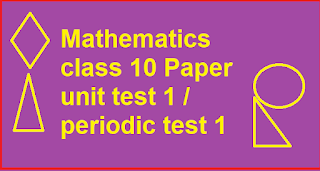Mathematics Class 10 Unit Test 1 / Periodic Test 1 Sample paper - GovtJobsInfo.in

Always Type <== GovtJobsInfo.In ==>Without Any Space in Google for this website

## Tuesday, May 30, 2023Class 10  maths unit test 1 periodic test 1 question paper

periodic test 1 question paper class 10 math

### SUBJECT: MATHEMATICS             MAX. MARKS : 10

CLASS : X                                                       DURATION : 1 HRS### General Instructions:

(i).         All questions are compulsory.

(ii).       This question paper contains 11 questions divided into three Sections A, B and C.

(iii).     Section A comprises of 04 questions of 1/2 mark each. Section B comprises of 06 questions of 1 marks each. Section C comprises of 01 question of 2 marks .# Questions 1 to 4 carry 1/2 mark each.

1.    If two positive integers a and b are written as a = x5y3 and b = xy2 ; x, y are prime numbers, then HCF (a, b) is

(A)xy                           (B)xy2              (C)x3y3                        (D)x2y2

2.    The least number that is divisible by all the numbers from 1 to 10 (both inclusive) is

(A)10                           (B)100             (C)504             (D)2520

3.    The product of a non-zero rational and an irrational number is:

(A)  always irrational (B) always rational (C) rational or irrational (D) one

4.    The zeroes of the quadratic polynomial x2 + 99x + 127 are

(A)   both positive        (B) both negative                    (C)   one positive and one negative

(D) both equal

# Questions 5 to 8 carry 1 mark each.

5.    The numbers 525 and 3000 are both divisible only by 3, 5, 15, 25 and 75. What is HCF (525, 3000)? Justify your answer

6.    Prove that root(7)+ 5 is irrational.

7.    Find the zeroes of the following quadratic polynomial and verify the relationship between the zeroes and the coefficients: 5t 2 + 12t + 7.

8.    Find a quadratic polynomial each with the given numbers as the sum and product of its zeroes respectively: root(3) and 1/3.

9.    Half the perimeter of a rectangular garden, whose length is 4 m more than its width, is 36 m. Find the dimensions of the garden.

10.  Solve 2x + 3y = 11 and 2x – 4y = – 24 and hence find the value of ‘m’ for which y = mx + 3.

SECTION C

Question 11  carry 2 marks

11.   Solve the following pair of linear equations by the elimination method:  3x-5y-4=0 , 9x =

# 2y +7

----------X----------